Anúncio

# Unmanaged Parallelization via P/Invoke

22 de Sep de 2009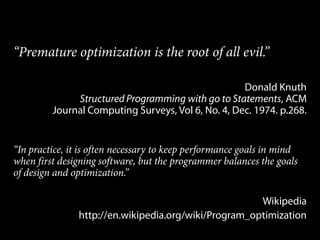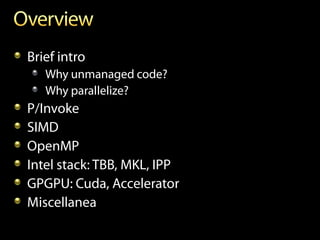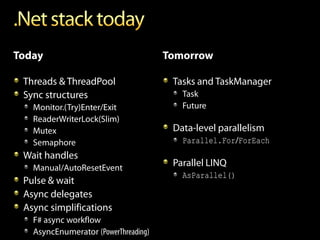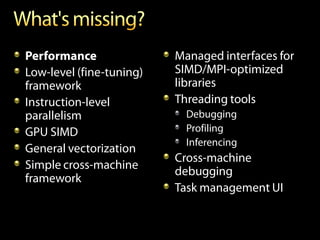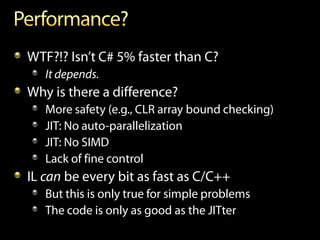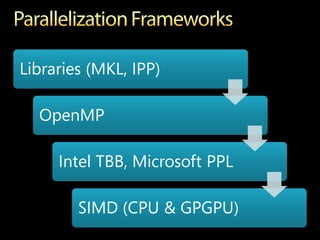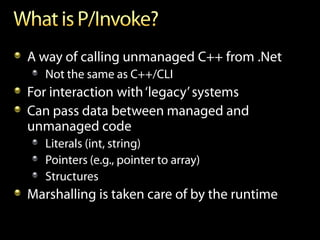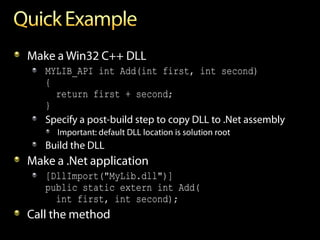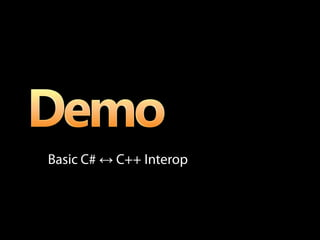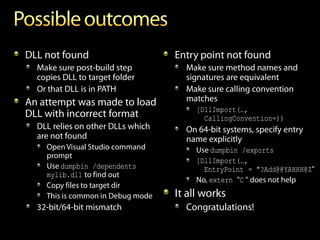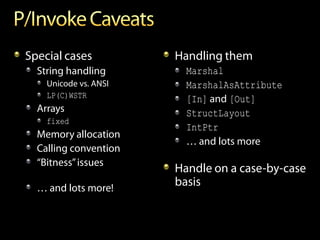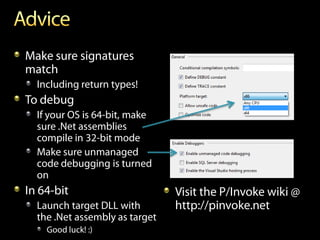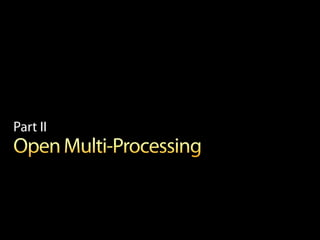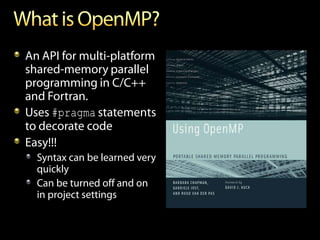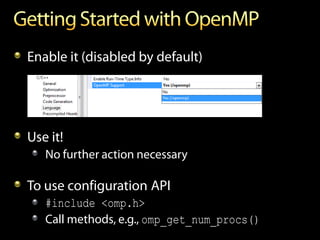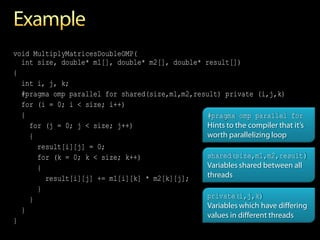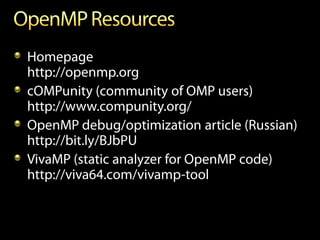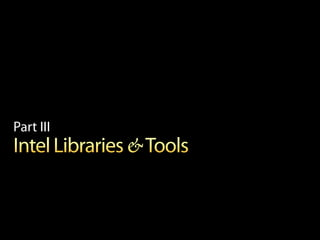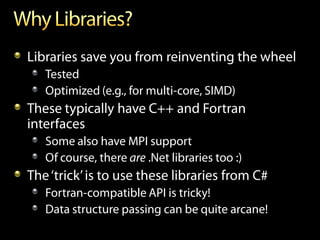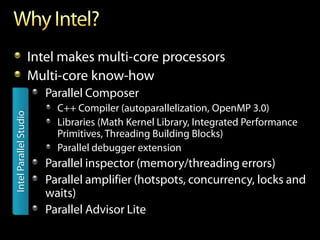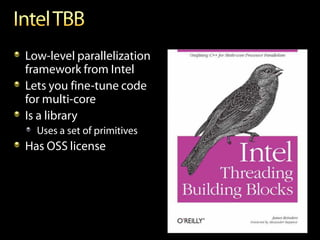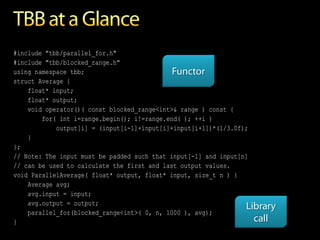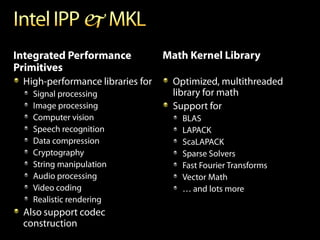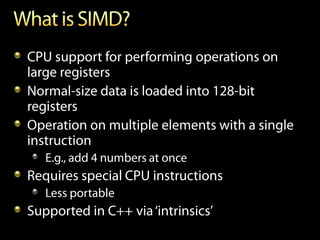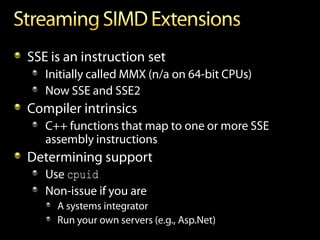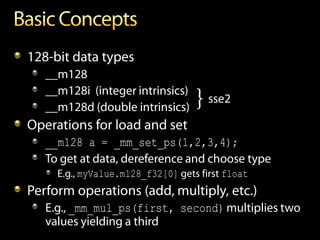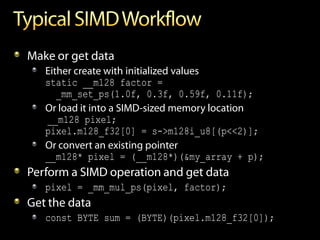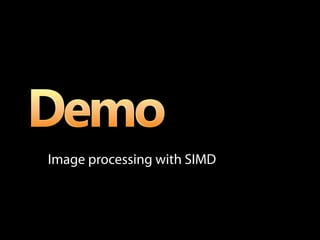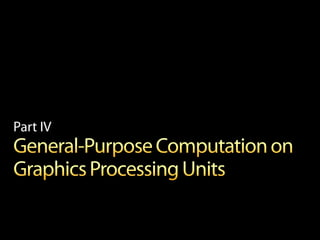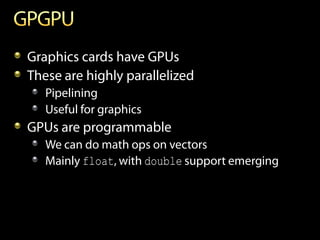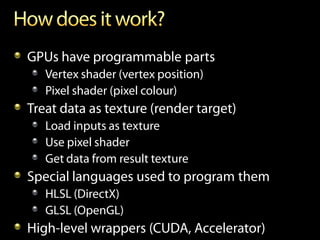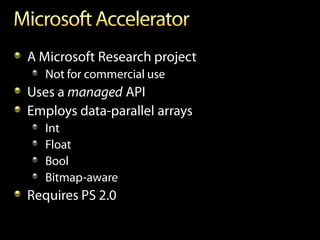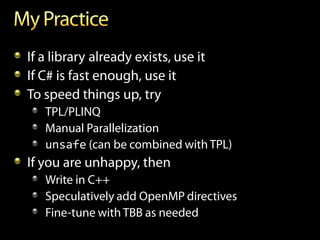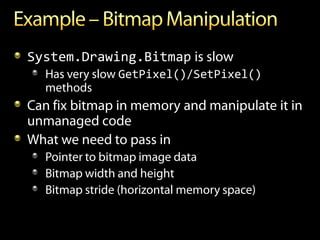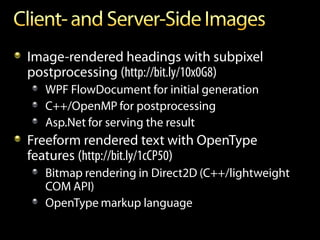1 de 42
Anúncio

### Unmanaged Parallelization via P/Invoke

1. Dmitri Nesteruk dmitri@activemesa.com http://activemesa.com http://spbalt.net http://devtalk.net
2. “Premature optimization is the root of all evil.” Donald Knuth Structured Programming with go to Statements, ACM Journal Computing Surveys, Vol 6, No. 4, Dec. 1974. p.268. “In practice, it is often necessary to keep performance goals in mind when first designing software, but the programmer balances the goals of design and optimization.” Wikipedia http://en.wikipedia.org/wiki/Program_optimization
3. Brief intro Why unmanaged code? Why parallelize? P/Invoke SIMD OpenMP Intel stack: TBB, MKL, IPP GPGPU: Cuda, Accelerator Miscellanea
5. Performance Managed interfaces for Low-level (fine-tuning) SIMD/MPI-optimized framework libraries Instruction-level Threading tools parallelism Debugging GPU SIMD Profiling Inferencing General vectorization Cross-machine Simple cross-machine debugging framework Task management UI
6. WTF?!? Isn’t C# 5% faster than C? It depends. Why is there a difference? More safety (e.g., CLR array bound checking) JIT: No auto-parallelization JIT: No SIMD Lack of fine control IL can be every bit as fast as C/C++ But this is only true for simple problems The code is only as good as the JITter
7. Libraries (MKL, IPP) OpenMP Intel TBB, Microsoft PPL SIMD (CPU & GPGPU)
8. Part I
9. A way of calling unmanaged C++ from .Net Not the same as C++/CLI For interaction with ‘legacy’ systems Can pass data between managed and unmanaged code Literals (int, string) Pointers (e.g., pointer to array) Structures Marshalling is taken care of by the runtime
10. Make a Win32 C++ DLL MYLIB_API int Add(int first, int second) { return first + second; } Specify a post-build step to copy DLL to .Net assembly Important: default DLL location is solution root Build the DLL Make a .Net application [DllImport("MyLib.dll")] public static extern int Add( int first, int second); Call the method
11. Basic C# ↔ C++ Interop
12. DLL not found Entry point not found Make sure post-build step Make sure method names and copies DLL to target folder signatures are equivalent Or that DLL is in PATH Make sure calling convention An attempt was made to load matches [DllImport(…, DLL with incorrect format CallingConvention=)) DLL relies on other DLLs which On 64-bit systems, specify entry are not found name explicitly Open Visual Studio command Use dumpbin /exports prompt [DllImport(…, Use dumpbin /dependents EntryPoint = "?Add@@YAHHH@Z" mylib.dll to find out No, extern "C " does not help Copy files to target dir This is common in Debug mode It all works 32-bit/64-bit mismatch Congratulations!
13. Special cases Handling them String handling Marshal Unicode vs. ANSI MarshalAsAttribute LP(C)WSTR [In] and [Out] Arrays StructLayout fixed IntPtr Memory allocation … and lots more Calling convention “Bitness” issues Handle on a case-by-case … and lots more! basis
14. Make sure signatures match Including return types! To debug If your OS is 64-bit, make sure .Net assemblies compile in 32-bit mode Make sure unmanaged code debugging is turned on In 64-bit Visit the P/Invoke wiki @ Launch target DLL with http://pinvoke.net the .Net assembly as target Good luck! :)
15. Part II
16. An API for multi-platform shared-memory parallel programming in C/C++ and Fortran. Uses #pragma statements to decorate code Easy!!! Syntax can be learned very quickly Can be turned off and on in project settings
17. Enable it (disabled by default) Use it! No further action necessary To use configuration API #include <omp.h> Call methods, e.g., omp_get_num_procs()
18. void MultiplyMatricesDoubleOMP( int size, double* m1[], double* m2[], double* result[]) { int i, j, k; #pragma omp parallel for shared(size,m1,m2,result) private (i,j,k) for (i = 0; i < size; i++) { #pragma omp parallel for for (j = 0; j < size; j++) Hints to the compiler that it’s { worth parallelizing loop result[i][j] = 0; for (k = 0; k < size; k++) shared(size,m1,m2,result) { Variables shared between all result[i][j] += m1[i][k] * m2[k][j]; threads } } private(i,j,k) Variables which have differing } values in different threads }
19. Using OpenMP in your C++ app
20. Homepage http://openmp.org cOMPunity (community of OMP users) http://www.compunity.org/ OpenMP debug/optimization article (Russian) http://bit.ly/BJbPU VivaMP (static analyzer for OpenMP code) http://viva64.com/vivamp-tool
21. Part III
22. Libraries save you from reinventing the wheel Tested Optimized (e.g., for multi-core, SIMD) These typically have C++ and Fortran interfaces Some also have MPI support Of course, there are .Net libraries too :) The ‘trick’ is to use these libraries from C# Fortran-compatible API is tricky! Data structure passing can be quite arcane!
23. Intel makes multi-core processors Multi-core know-how Parallel Composer C++ Compiler (autoparallelization, OpenMP 3.0) Intel Parallel Studio Libraries (Math Kernel Library, Integrated Performance Primitives, Threading Building Blocks) Parallel debugger extension Parallel inspector (memory/threading errors) Parallel amplifier (hotspots, concurrency, locks and waits) Parallel Advisor Lite
24. Low-level parallelization framework from Intel Lets you fine-tune code for multi-core Is a library Uses a set of primitives Has OSS license
25. #include "tbb/parallel_for.h" #include "tbb/blocked_range.h" using namespace tbb; Functor struct Average { float* input; float* output; void operator()( const blocked_range<int>& range ) const { for( int i=range.begin(); i!=range.end( ); ++i ) output[i] = (input[i-1]+input[i]+input[i+1])*(1/3.0f); } }; // Note: The input must be padded such that input[-1] and input[n] // can be used to calculate the first and last output values. void ParallelAverage( float* output, float* input, size_t n ) { Average avg; avg.input = input; avg.output = output; Library parallel_for(blocked_range<int>( 0, n, 1000 ), avg); } call
26. Integrated Performance Math Kernel Library Primitives High-performance libraries for Optimized, multithreaded Signal processing library for math Image processing Support for Computer vision BLAS Speech recognition LAPACK Data compression ScaLAPACK Cryptography Sparse Solvers String manipulation Fast Fourier Transforms Audio processing Vector Math Video coding … and lots more Realistic rendering Also support codec construction
27. Part IV
28. CPU support for performing operations on large registers Normal-size data is loaded into 128-bit registers Operation on multiple elements with a single instruction E.g., add 4 numbers at once Requires special CPU instructions Less portable Supported in C++ via ‘intrinsics’
29. SSE is an instruction set Initially called MMX (n/a on 64-bit CPUs) Now SSE and SSE2 Compiler intrinsics C++ functions that map to one or more SSE assembly instructions Determining support Use cpuid Non-issue if you are A systems integrator Run your own servers (e.g., Asp.Net)
30. 128-bit data types __m128 __m128i (integer intrinsics) __m128d (double intrinsics) } sse2 Operations for load and set __m128 a = _mm_set_ps(1,2,3,4); To get at data, dereference and choose type E.g., myValue.m128_f32 gets first float Perform operations (add, multiply, etc.) E.g., _mm_mul_ps(first, second) multiplies two values yielding a third
31. Make or get data Either create with initialized values static __m128 factor = _mm_set_ps(1.0f, 0.3f, 0.59f, 0.11f); Or load it into a SIMD-sized memory location __m128 pixel; pixel.m128_f32 = s->m128i_u8[(p<<2)]; Or convert an existing pointer __m128* pixel = (__m128*)(&my_array + p); Perform a SIMD operation and get data pixel = _mm_mul_ps(pixel, factor); Get the data const BYTE sum = (BYTE)(pixel.m128_f32);
32. Image processing with SIMD
33. Part IV
34. Graphics cards have GPUs These are highly parallelized Pipelining Useful for graphics GPUs are programmable We can do math ops on vectors Mainly float, with double support emerging
35. GPUs have programmable parts Vertex shader (vertex position) Pixel shader (pixel colour) Treat data as texture (render target) Load inputs as texture Use pixel shader Get data from result texture Special languages used to program them HLSL (DirectX) GLSL (OpenGL) High-level wrappers (CUDA, Accelerator)
36. A Microsoft Research project Not for commercial use Uses a managed API Employs data-parallel arrays Int Float Bool Bitmap-aware Requires PS 2.0
37. Sorry! No demo. Accelerator does not work on 64-bit :(
38. If a library already exists, use it If C# is fast enough, use it To speed things up, try TPL/PLINQ Manual Parallelization unsafe (can be combined with TPL) If you are unhappy, then Write in C++ Speculatively add OpenMP directives Fine-tune with TBB as needed
39. System.Drawing.Bitmap is slow Has very slow GetPixel()/SetPixel() methods Can fix bitmap in memory and manipulate it in unmanaged code What we need to pass in Pointer to bitmap image data Bitmap width and height Bitmap stride (horizontal memory space)
40. Image-rendered headings with subpixel postprocessing (http://bit.ly/10x0G8) WPF FlowDocument for initial generation C++/OpenMP for postprocessing Asp.Net for serving the result Freeform rendered text with OpenType features (http://bit.ly/1cCP50) Bitmap rendering in Direct2D (C++/lightweight COM API) OpenType markup language
41. a l b v o b q l l k u t m y w m w r e e r q q m q i q d n w g s s w d a v p d v n u x j l s y t u b n b y c t h r r y u v a s t a d t n z f f x g q h b j j p y o w s i g i c i i g s o f n f r j f d c f g m k w u y j v b v e m i t i j x u v w s j u g u y l b o c m y k u b w s w n p x i o k a y c q o s u n k s c g x j x j e q p h j i a c m j z h c k v x k a k f e c r u u x q p p k o f w g x b v j m b e l e e w k s c v n n o g c z w w f w i n e h j q l h x u v j o m h g s x a j z b d n u a s c n a j i x w i n w z j d s p n w i p c n d s r m j h z q j g b w j m e z k j v a z o u q w d c j c f o x w t h v s r h o m j y n a u p p u p h z n s j r m b z o w k i n t h l i k z w m z m f x c h o m w x b s m x u c j x o s h x u e t p u x e o v l h a y p f f v a x z x l z u l c l n q g e g m x y k k k q j n h p i j w i p d d a x z s z e m p c l i m s u g e i z o m q p r p d w m y q t o v m p T H E y E N D v z d c z x m g q q r h n b j i b q i p x n h w i d o h m a w c x m g h c y r i k n p n d m c x l z e h h s c l f s y l k j s p t d q e b k v u x k m k z p g k e n a f h h r o x v w k u j u t n e u q f a d n e d y y y f c z c a p x y f b r w e y o f a v f h z r y a n z u q r o g n f p x l j y l u a n r d o r v k m f j y n h p c c t k x y t b f j r n x g c z h s p c e i q g x k p f g r n l y i i f t i s b i f c k c h e s l w y s u p d v x b r l q l k i z d z w s a w r i i u m n i x r c j n d h n w g s f s i l h a b h l h x m v p t e g k n o i s g s x v b o k e c i j y b e d r t p e x v r c w u v d s d o a z t t m u i u v u b p l w c p x n k k v a a v b b s e e f d b f y i v c j k r g r y t j a m f v h b f s b z l i n a x c l r l z i v l c b n u d l l g u y r t t u q t l y j l q u h a o u o p t g v l q q r k r q y p l z d x n q n q v t f b u h r y n k f q i t h i u w i n m l o c c c
Anúncio